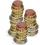# Prime numbers + multiplication principle - examples

1. No. of divisorsHow many different divisors has number ??
2. RectanglesHow many rectangles with area 3032 cm2 whose sides is natural numbers are?
3. StacksAnnie has a total of \$ 702. The money must be divided into stacks so that each buyer has the same amounth of dollars. How many options she have?
4. CombinationsHow many different combinations of two-digit number divisible by 4 arises from the digits 3, 5 and 7?
5. Inverted nineIn the hotel,, Inverted nine" each hotel room number is divisible by 6. How many rooms we can count with three-digit number registered by digits 1,8,7,4,9?

We apologize, but in this category are not a lot of examples.
Do you have an interesting mathematical example that you can't solve it? Enter it, and we can try to solve it.

To this e-mail address, we will reply solution; solved examples are also published here. Please enter e-mail correctly and check whether you don't have a full mailbox.